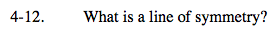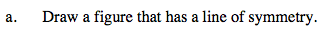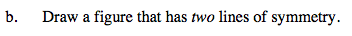### Home > A2C > Chapter 4 > Lesson 4.1.1 > Problem4-12

4-12.
1. What is a line of symmetry? 4-12 HW eTool (Desmos). Homework Help ✎

1. Draw a figure that has a line of symmetry.

2. Draw a figure that has two lines of symmetry.

3. Can you find a basic geometric shape that has an infinite number of lines of symmetry?Use the eTool below to view examples of figures described in parts (a) (b) and (c).
Click the link at right for the full version of the eTool: A2C 4-12 HW eTool